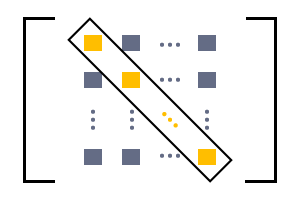# Diagonal elements of a Square Matrix

The elements which lie on the principal diagonal of a square matrix are called diagonal elements of a square matrix.Diagonal elements

Elements lie on the leading diagonal of a square matrix and these elements are known as diagonal elements of the matrix. In the case of second order square matrix, the first element of first row and the last element of last row are the diagonal elements of the square matrix.

In the case of higher than the second order square matrices, not only the first element of first row and last element of last row are known as diagonal elements and there are other elements which lie on the leading diagonal, are also called diagonal elements of the square matrix.

$M=\left[\begin{array}{ccccc}{e}_{1⁣1}& {e}_{1⁣2}& {e}_{1⁣3}& \cdots & {e}_{1⁣n}\\ {e}_{2⁣1}& {e}_{2⁣2}& {e}_{2⁣3}& \cdots & {e}_{2⁣n}\\ {e}_{3⁣1}& {e}_{3⁣2}& {e}_{3⁣3}& \cdots & {e}_{3⁣n}\\ ⋮& ⋮& ⋮& \ddots & ⋮\\ {e}_{n⁣1}& {e}_{n⁣2}& {e}_{n⁣3}& \cdots & {e}_{n⁣n}\end{array}\right]$

The elements ${e}_{11}$ and ${e}_{nn}$ are first element of first row and last element of last row of the square matrix. They are diagonal elements. Not only ${e}_{11}$ and ${e}_{nn}$, the elements ${e}_{22},{e}_{33},{e}_{44}\dots$ also lie on the square matrix. Therefore, the elements ${e}_{11},{e}_{22},{e}_{33}$ and up to ${e}_{nn}$ lie on the leading diagonal. Therefore, they are called diagonal elements of the matrix

## Example

$A$ is a fifth order square matrix. $25$ elements are arranged in $5$ rows and $5$ columns.

$A=\left[\begin{array}{ccccc}8& 5& 3& 9& 0\\ 3& 5& –5& 9& 2\\ 4& 2& 1& 0& 6\\ 2& 3& 2& 6& 7\\ 1& 9& 0& 3& 4\end{array}\right]$

In this ${A}_{5×5}$ square matrix, the elements $8,5,1,6$ and $4$ lie on the leading diagonal of the square matrix. Therefore, the elements $8,5,1,6$ and $4$ are called diagonal elements of matrix A.

Latest Math Topics
Latest Math Problems
Email subscription
Math Doubts is a best place to learn mathematics and from basics to advanced scientific level for students, teachers and researchers. Know more